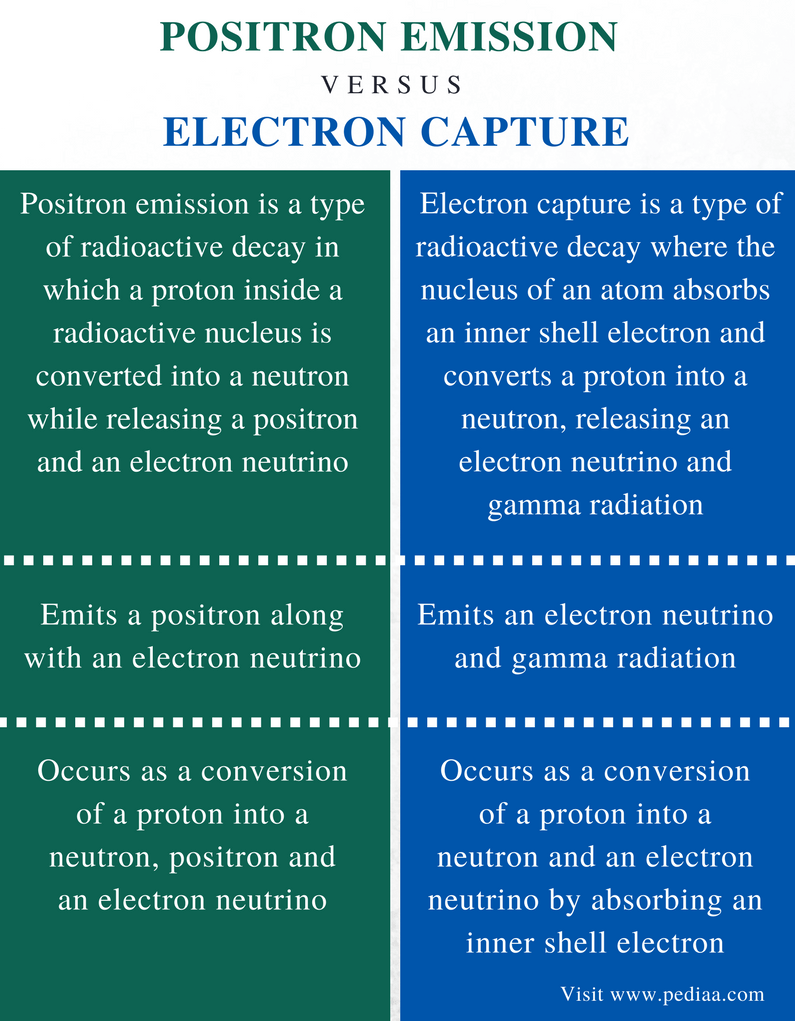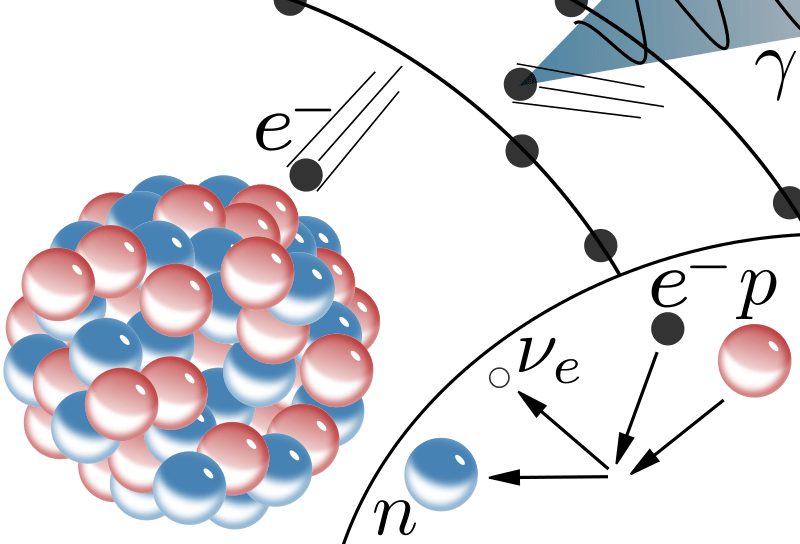# Difference Between Positron Emission and Electron Capture

## Main Difference – Positron Emission vs Electron Capture

There are certain naturally occurring isotopes that are unstable due to the imbalanced numbers of protons and neutrons they have in their nucleus of atoms. Therefore, in order to become stable, these isotopes undergo a spontaneous process called radioactive decay. Radioactive decay causes an isotope of a particular element to be converted into an isotope of a different element. There are different decay pathways such as positron emission, negatron emission and electron capture. Positron emission is the release of a positron and an electron neutrino in the process of radioactive decay. Electron capture is a process which emits an electron neutrino. Both these processes take place in proton-rich nuclei. In positron emission, a proton inside the radioactive nucleus is converted into a neutron while releasing a positron; in electron capture, a proton-rich nucleus of a neutral atom absorbs an inner shell electron which then converts a proton into a neutron, emitting an electron neutrino. This is the main difference between positron emission and electron capture.

### Key Areas Covered

1. What is Positron Emission
– Definition, Principle, Example
2. What is Electron Capture
– Definition, Principle, Example
3. What are the Similarities Between Positron Emission and Electron Capture
– Outline of Common Features
4. What is the Difference Between Positron Emission and Electron Capture
– Comparison of Key Differences

Key Terms: Atom, Electron, Electron Neutrino, Nucleus, Neutron, Positron, Proton, Radioactive Decay## What is Positron Emission

Positron emission is a type of radioactive decay where a proton inside a radioactive nucleus is converted into a neutron while releasing a positron and an electron neutrino. This is also known as beta plus decay. A positron is a subatomic particle with the same mass as an electron and a numerically equal but positive charge. It is also called beta particle+ or e+). An electron neutrino (Ve) is a subatomic particle that has no net electrical charge. The positron emission takes place in proton-rich radioactive nuclei.Figure 1: Positron Emission in a Diagram

In positron emission, the atomic number of the nucleus is decreased by 1. The atomic number of an atom is the total number of protons present in the nucleus. But in positron emission, one of these protons undergo a conversion. It causes the reduction of the atomic number. However, the mass number of the atom will remain the same. This is because the proton is converted into a neutron and the mass number is the sum of protons and neutrons in the atom. Following nuclear reaction is an example of positron emission.

611C   →    511B  +   e+    +    Ve    +   energy

This is an isotope of carbon. It is a radioactive isotope of carbon. It decays to boron-11 via positron emission. Boron-11 is a stable isotope of boron.

## What is Electron Capture

Electron capture is a type of radioactive decay where the nucleus of an atom absorbs an inner shell electron and converts a proton into a neutron releasing an electron neutrino and gamma radiation. This process takes place in proton-rich nuclei. An inner shell electron is an electron from an inner energy level of the atom (ex: K shell, L shell). Simultaneously, this process causes the release of an electron neutrino. The nuclear reaction for the process can be given as follows.

P   +   e    →     n    +    Ve  +   γFigure 2: Electron Capture Principle

Electron capture causes the reduction of an atomic number by 1 because the atomic number is the total number of protons in an atomic nucleus, and in this process, a proton undergoes conversion into a neutron. However, the mass number does not change. Since the electron capture results in a loss of an electron in the electron shell, it is balanced by the loss of a proton (positive charge), hence the atom remains electrically neutral.

13N7 + e13C6 + Ve + γ

The above reaction gives the electron capture of a nitrogen isotope. It forms a carbon-13 atom along with an electron neutrino and gamma radiation. Carbon-13 is a natural, stable isotope of carbon.

## Similarities Between Positron Emission and Electron Capture

• Both are forms of radioactive decay.
• Both forms take place in proton-rich
• Both form release electron neutrino.
• Both forms do not change the atomic number or mass number of an atom.

## Difference Between Positron Emission and Electron Capture

### Definition

Positron Emission: Positron emission is a type of radioactive decay in which a proton inside a radioactive nucleus is converted into a neutron while releasing a positron and an electron neutrino.

Electron Capture: Electron capture is a type of radioactive decay in which the nucleus of an atom absorbs an inner shell electron and converts a proton into a neutron, releasing an electron neutrino and gamma radiation.

### Emission

Positron Emission: Positron emission emits a positron along with an electron neutrino.

Electron Capture: Electron capture emits an electron neutrino and gamma radiation.

### Principle

Positron Emission: Positron emission occurs as a conversion of a proton into a neutron, positron and an electron neutrino.

Electron Capture: Electron capture occurs as a conversion of a proton into a neutron and an electron neutrino by absorbing an inner shell electron.

### Conclusion

Radioactive decay of an unstable isotope of a particular element converts that isotope into a different isotope of a different chemical element. There are several decay pathways. Positron emission and electron capture are two such pathways. The main difference between positron emission and electron capture is that, in positron emission, a proton inside the radioactive nucleus is converted into a neutron while releasing a positron whereas, in electron capture, a proton-rich nucleus of a neutral atom absorbs an inner shell electron which then converts a proton into a neutron emitting an electron neutrino.

##### Reference:

1. Helmenstine, Anne Marie. “Electron Capture Definition.” ThoughtCo, Jun. 25, 2014, Available here.
2. “Decay Pathways.” Chemistry LibreTexts, Libretexts, 10 June 2017, Available here.

##### Image Courtesy:

1. “Beta-plus Decay” By Master-m1000 – Own work based on: Beta-minus Decay.svg  by Inductiveload (Public Domain) via Commons Wikimedia
2. “Electron capture” By Master-m1000 – and self-made. This vector image was created with Inkscape (Public Domain) via Commons Wikimedia